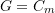### Centres of Bodies: Center of mass

In some engineering analysis, we may need to consider the mass of an object. For example, center of mass appears in dynamic equations of motion. It is proved that applying a force to the center of mass of a body causes the body to accelerate along a line without developing angular (rotational) acceleration.

Similar to the center of gravity, the distribution of mass of a body can also be simplified and represented by a total mass at a particular point called the center of mass. The location of the center of mass (of a body) is determined by a mathematical definition expressing the center of mass as the average/mean location of the spatial distribution of the mass of the body.

For a system with n particles each having a mass of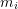and located in the space with coordinates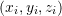, as shown in Fig. 9.4a. The center of mass,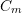, of the system has the following coordinates

(9.10)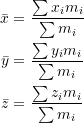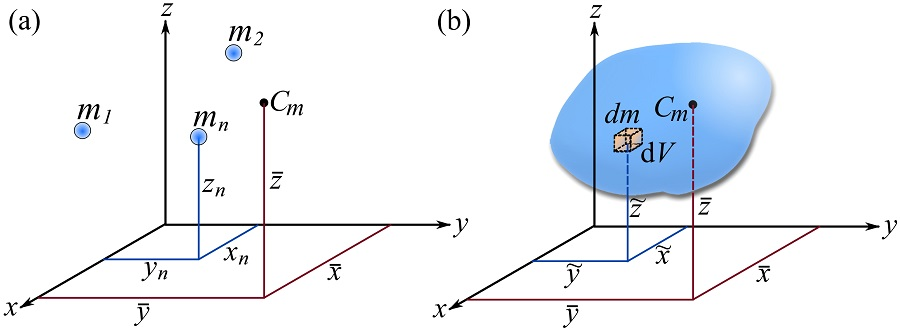Fig. 9.4 (a) Center of mass of a set of discrete particles, (b) center of mass of a continuous body.

where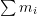is the total mass of the particles.

The center of mass of a continuous body (Fig. 9.4b), can be determined in a way similar to that for the center of gravity (see Eq. in Section ) can be determined using Eqs 9.11

(9.11)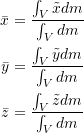where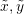and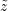are the coordinates of the center of mass of the volumetric differential element. Note that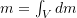is the total mass of the body.

By considering the material density,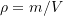where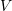is the volume, and writing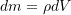, Eqs. 9.11 become,

(9.12)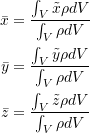#### The relationship between the centers of gravity and mass

The center of gravity depends on the (vector) distribution of the gravitational field over the points of a body while the center of mass depends on the distribution of the mass of the body. On Earth, where the bodies considered in this course are far smaller than Earth, the gravitational field can be considered uniform on the bodies and pointing perpendicularly downward (i.e., in the same direction). Therefore, the weight and mass of the body can be related by a scalar formula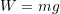. Substitution of this relation in the equations of center of gravity, results in the equality of the center of mass and the center of gravity of bodies on Earth,

(9.13)